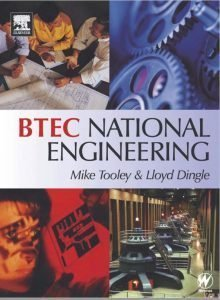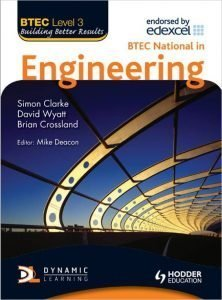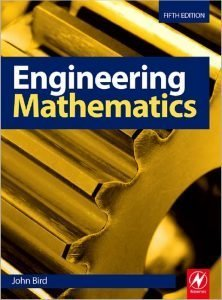# Mathematics for Engineering Technicians

£275.00

Level 3 Maths is needed and should be studied before any Principles Units.

You will have 2 months to complete this as a stand alone unit

SKU: A/600/0253 Category:

# Mathematics for Engineering Technicians

### Aim and purpose

This unit aims to give learners a strong foundation in mathematical skills. These skills will help them to successfully complete many of the other units within the qualification.

### Unit introduction

One of the main responsibilities of engineers is to solve problems quickly and effectively. This unit will enable learners to solve mathematical, scientific and associated engineering problems at technician level. It will also act as a basis for progression to study other units both within the qualification, such as Unit 28: Further Mathematics for Technicians, and at BTEC Higher National level.

This unit enables learners to build on knowledge gained at GCSE or BTEC First Diploma level and use it in a more practical context for their chosen discipline. Learning outcome 1 will develop learners’ knowledge and understanding of algebraic methods, from a look at the use of indices in engineering to the use of the algebraic formula for solving quadratic equations. Learning outcome 2 involves the introduction of the radian as another method of angle measurement, the shape of the trigonometric ratios and the use of standard formulae to solve surface areas and volumes of regular solids. Learning outcome 3 requires learners to be able to represent statistical data in a variety of ways and calculate the mean, median and mode. Finally, learning outcome 4 is intended as a basic introduction to the arithmetic of elementary calculus.

### Learning outcomes

On completion of this unit a learner should:

1. Be able to use algebraic methods
2. Be able to use trigonometric methods and standard formula to determine areas
3. Be able to use statistical methods to display data
4. Be able to use elementary calculus techniques.

This unit is a mandatory unit in all the Level 3 Engineering courses, however can be taken as an individual unit. Especially if you are planning to study Higher National Engineering.

### Included Books (available as pdf’s)